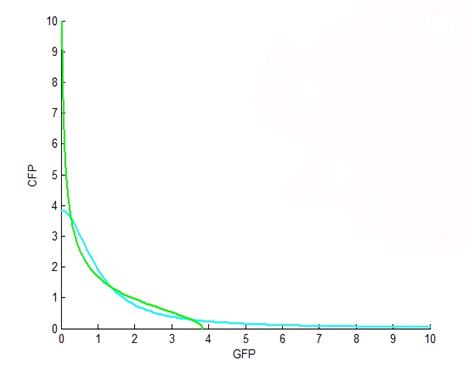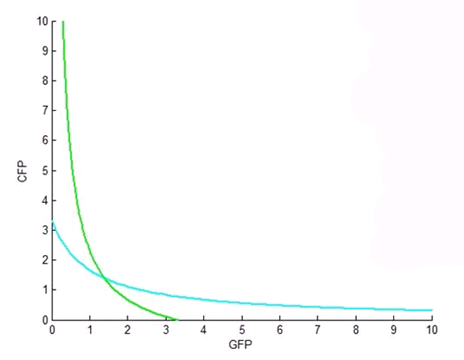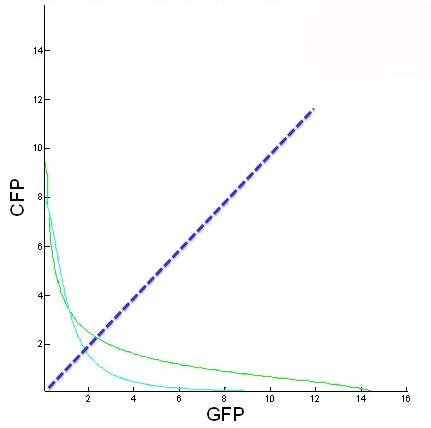# Team:Aberdeen Scotland/Fixed Points

(Difference between revisions)
 Revision as of 16:07, 27 October 2010 (view source)Brychan (Talk | contribs)← Older edit Revision as of 16:07, 27 October 2010 (view source)Brychan (Talk | contribs) Newer edit → Line 54: Line 54:

- -

Figure 3. Figure 3: This figure is of CFP as a function of GFP for the two nullclines when the initial galactose and copper concentrations are changed. From this we can see how the fixed points change with different initial galactose and methionine concentrations. The blue line represents the GFP = CFP line. The further from this line equilibrium points are, the harder it will be to switch between stable states.

Figure 3. Figure 3: This figure is of CFP as a function of GFP for the two nullclines when the initial galactose and copper concentrations are changed. From this we can see how the fixed points change with different initial galactose and methionine concentrations. The blue line represents the GFP = CFP line. The further from this line equilibrium points are, the harder it will be to switch between stable states.

## Revision as of 16:07, 27 October 2010

University of Aberdeen - ayeSwitch - iGEM 2010

iGEM 2010

# Fixed Points

Fixed points are the points where an equation’s rate of change, or its slope, is zero. There are three main types of equilibrium points: stable, unstable, and saddle-node points. A stable equilibrium is a value which the function converges towards, whereas an unstable equilibrium is a value the function will diverge from. A saddle-node is where the function both converges to and diverges from, the equilibrium, depending on the direction from which we approach the point (S. Strogatz, Nonlinear dynamics and Chaos).

### Why are fixed points important?

Finding fixed points is the first step in analyzing the stability of a system. In particular, we are interested in the nature of our system’s bistability and how it changes with a variation of the parameters, i.e., its bifurcation analysis. This analysis can be conveyed to the biologists to minimize experimental guessing.

### How are fixed points calculated?

Fixed points are determined by setting all differential equations in a system equal to zero and solving for the variable being analyzed. The direct method of calculating equilibrium points is to find the roots of the system of equations using built-in root-finding functions such as fzero in MATLAB, and similar functions in Maple and C. The indirect method of calculating the equilibrium points is to plot and find the intersections of these nullclines, which represent equilibrium points.

### Results

We were able to find the fixed points of the system analytically (for small Hill coefficients) and computationally. These points were used for bifurcation analysis, and for analyzing the probability that our system would exhibit bistable behavior.

# Nullclines

In a system of differential equations, the nullclines are the solution curves for which all of the differential equations are equal to zero (Wikipedia).

### Why are nullclines important?

The intersections of the nullclines give the equilibrium points of the system of differential equations. From graphs of the nullclines, it is possible to infer whether or not a system will be bistable. If the nullclines only intersect in one place the system is not bistable, since there is one single equilibrium point. If there are more than two intersections, the middle equilibrium point is often an unstable saddle point.

### How are the nullclines calculated?

Just as in calculating the fixed points, we set the governing differential equations of the system equal to zero and plot the curves generated.

### Simulations involved

Just as in calculating the equilibrium points, we set the governing differential equations of the system equal to zero and plot the curves generated.

equationsFigure 1.This figure is a plot of the nullclines of the differential equations for CFP and GFP, where we solve them for CFP as a function of GFP. In this plot, both Hill coefficients are two, ie. n2 and n4 are both 2. The nullclines cross over at three fixed points, where the middle is a saddle-node fixed point. This is an ‘ideal’ bistability plot.Figure 2.This figure is a plot, again, of the nullclines of the differential equations for CFP and GFP. In this plot, both Hill coefficients are one. Note that the nullclines only cross once, resulting in only one fixed point and hence no possibility of bistability. This result allowed us to tell the biologists that the toggle switch would definitely not work if both of the Hill coefficients were one.Figure 3. Figure 3: This figure is of CFP as a function of GFP for the two nullclines when the initial galactose and copper concentrations are changed. From this we can see how the fixed points change with different initial galactose and methionine concentrations. The blue line represents the GFP = CFP line. The further from this line equilibrium points are, the harder it will be to switch between stable states.

### Results

We focused our efforts on plotting the nullclines of the system for a range of Hill coefficient combinations in order to get a general idea of which combinations would most likely produce robust bistability. We found that all combinations gave bistability, except when both Hill coefficients were one. The optimal Hill coefficient combination occurred when both Hill coefficients were two. We passed this information onto the biology team, letting them know that if we wanted the system to successfully switch, we could not have both Hill coefficients with a value of one.

Back to the Top